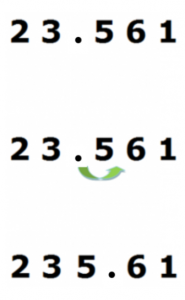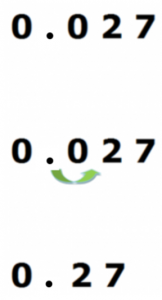Smartick is a fun way to learn math!Sep27

# Learn to Multiply a Decimal by 10

Do you know how to multiply a decimal number by 10? In this post, we will explain the easiest way to do so.

To multiply a decimal number by 10, we just have to move the decimal point one position to the right.

Yes, folks, it’s that easy.

### Example #1:

23.561 x 10

We have to move the decimal point one position to the right:The result of the multiplication is 235.61.

### Example #2

0.027 x 10

We move the decimal point one position to the right:The result of the multiplication is 0.27.
See how easy! Did you like this post? Share it with your friends so they can also learn to multiply a decimal number by 10.

Also, you can register in Smartick to learn much more math!